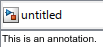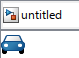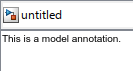## Create and Edit Annotations Programmatically

Annotations are visual elements that you can use to add descriptive notes and callouts to your model. In addition to text-only annotations, you can create annotations that:

• Open websites

• Perform MATLAB® commands

• Display images

• Visually differentiate areas of block diagrams

These examples show how to programmatically create, edit, and delete annotations.

### Create Annotation

This example shows how to create, modify, and view an annotation programmatically.

Open a new model.

`open_system(new_system)`

Create an annotation with default properties using the `Simulink.Annotation` function.

`a = Simulink.Annotation(gcs,'This is an annotation.');`After creating the annotation, use dot notation to set property values. For example, apply an 18-point font and yellow background to the annotation.

```a.FontSize = 18; a.BackgroundColor = 'yellow';```

To view and briefly highlight the new annotation, use the `view` function.

`view(a)`### Find and Modify Annotations

This example shows how to find the annotations in the `vdp` model and modify the properties of one of the annotations programmatically.

Open the example. Then, open the `vdp` model.

`open_system('vdp')`To find the annotations in the model, use the `find_system` function.

`h = find_system(gcs,'FindAll','on','Type','annotation');`

To identify the annotations, query the text inside the annotations by using the `get_param` function.

`get_param(h,'PlainText')`
```ans = 3x1 cell {'Copyright 2004-2022 The MathWorks, Inc.'} {'Van der Pol Equation' } {'x'' - µ(1-x^2) x' + x = 0' } ```

Suppose you want to apply a green background color to the `'Van der Pol Equation'` annotation.

Get the `Simulink.Annotation` object by specifying the corresponding index of the array.

`an = get_param(h(2),'Object');`

Use dot notation to set the value of the `BackgroundColor` property.

`an.BackgroundColor = 'Green';`### Delete Annotation

This example shows how to delete an annotation in the `vdp` model programmatically.

Open the example. Then, open the `vdp` model.

`open_system('vdp')`To get the handles for the annotations in the model, use the `find_system` function.

`h = find_system(gcs,'FindAll','on','Type','annotation');`

To identify the annotations, query the text inside the annotations.

`get_param(h,'PlainText')`
```ans = 3x1 cell {'Copyright 2004-2022 The MathWorks, Inc.'} {'Van der Pol Equation' } {'x'' - µ(1-x^2) x' + x = 0' } ```

To delete the title of the model, `'Van der Pol Equation'`, get the `Simulink.Annotation` object that corresponds to the second handle.

`an = get_param(h(2),'Object');`

Delete the annotation from the model.

`delete(an)`Get the handles for the annotations in the model. Then, query the text inside the annotations.

```h1 = find_system(gcs,'FindAll','on','Type','annotation'); get_param(h1,'PlainText')```
```ans = 2x1 cell {'Copyright 2004-2022 The MathWorks, Inc.'} {'x'' - µ(1-x^2) x' + x = 0' } ```

The model no longer has an annotation with `'Van der Pol Equation'` as text.

### Create Annotations That Contain Hyperlinks

This example shows how to use HTML formatting to add hyperlinks to text within rich-text annotations.

Open a new model.

`open_system(new_system)`

Create two annotations, moving one of the annotations so that it does not overlap the other.

```a1 = Simulink.Annotation(gcs,'This is an annotation.'); a2 = Simulink.Annotation(gcs,'This is another annotation.'); a2.Position = [0 20 28 34];```To create a hyperlink in the annotation, set `Interpreter` to `'rich'` and define the hyperlink in the `Text` property.

```a1.Interpreter = 'rich'; a1.Text = 'Go to <a href="www.mathworks.com">www.mathworks.com</a>.';```You can also embed MATLAB functions in the hyperlink.

```a2.Interpreter = 'rich'; a2.Text = '<a href="matlab:magic(4)">Generate magic square</a>.';```This example shows how to add an image to your model, such as a logo, by creating an image-only annotation programmatically.

Open the example. Then, open a new model and create an annotation in it.

```open_system(new_system) a = Simulink.Annotation(gcs,'This is an annotation.');```Change the annotation to display only the specified image.

```img = fullfile(pwd,'drivingCar_24.png'); setImage(a,img)```### Create Area

This examples shows how to create an area annotation in a model programmatically.

Open the example. Then, open the `vdp` model.

`open_system('vdp')`To create an area that includes some of the blocks in the model, use the `get_param` function to get the position of those blocks. For example, get the position of the blocks named `x1` and `x2`.

```x1_pos = get_param('vdp/x1','Position'); x2_pos = get_param('vdp/x2','Position');```

The function returns the position as a vector of coordinates, in pixels: `[left top right bottom]`. The origin is the upper-left corner of the Simulink Editor canvas before any canvas resizing. Positive values are to the right of and down from the origin. Negative values are to the left of and up from the origin.

To create an area around the blocks named `x1` and `x2`, use the `add_block` function with the `built-in/Area` option. Specify a relative position for the edges of the area based on the block positions.

```add_block('built-in/Area','vdp/This is an area',... 'Position',[x2_pos(1)-20,x2_pos(2)-20,x1_pos(3)+20,x1_pos(4)+20])```The area contains the blocks named `x1` and `x2`. Each side of the area is offset 20 pixels from the closest block edge.

### Create and Hide Markup Annotation

This example shows how to create markup annotations, which can be easily hidden.

Open a new model.

`open_system(new_system)`

Create two annotations, and move the second annotation so that it does not overlap the first annotation.

```a1 = Simulink.Annotation(gcs,'This is a model annotation.'); a2 = Simulink.Annotation(gcs,'This is another model annotation.'); a2.Position = [0 20 28 34];```By default, you create model annotations, which appear in the model.

Change the second annotation to a markup annotation.

```a2.MarkupType = 'markup'; a2.Text = 'This is a markup annotation.';```Configure the current model to hide markup annotations.

`set_param(gcs,'ShowMarkup','off');````ah = find_system(gcs,'FindAll','on','Type','annotation'); at = get_param(ah,'Text')```
```at = 2x1 cell {'This is a markup annotation.'} {'This is a model annotation.' } ```

Both annotations remain, despite the markup annotation being hidden.

### Find Annotation Executing Callback Function

If an annotation invoked a currently executing callback function, use the `getCallbackAnnotation` to determine which annotation invoked it. The function returns the corresponding `Annotation` object. This function is also useful if you write a callback function in a separate MATLAB file that contains multiple callback calls.